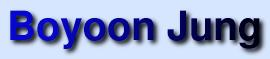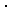Boyoon Jung's Library Manual

bj::KDCluster Class Reference [Machine Learning Techniques]

A fast data clustering algorithm using a kernel density tree. More...

#include <kd_cluster.h>

List of all members.

Public Member Functions

KDCluster (int dimension, double threshold, int max_clusters=10)
A constructor.
~KDCluster (void)
A destructor.
virtual int cluster (KDTree *tree)
Cluster data points using multiple gaussians.
int numClusters (void)
Return the number clusters.
gsl_vector * mean (int c)
Return the mean vector of a specific cluster.
gsl_matrix * covariance (int c)
Return the covariance matrix of a specific cluster.
double densityThreshold (void)
Return the density threshold.
void setDensityThreshold (double th)
Set a new density threshold.

Static Public Member Functions

void cov2ellipse (gsl_matrix *cov, double &w, double &h, double &a)
Convert a covariance matrix to an ellipsoidal parameters.

Protected Member Functions

double distance (gsl_vector *x, gsl_vector *y)
Compute the manhattan distance between two nodes.
void select_candidates (kd_node *tree, int npoints, kd_node **candidates, int &size)
Select candidate nodes from a kd-tree.

Protected Attributes

int nclusters
Number of clusters.
gsl_vector ** m
Mean vectors.
gsl_matrix ** cov
Covariance matrices.
int dimension
The dimension of input points.
int max_clusters
Maximum number of clusters.
double threshold
Density threshold.
gsl_vector * offsetv
Offset of a mean vector.
gsl_vector * mv
New mean vector.
gsl_vector * nmv
Newly introduced mean vector.
gsl_matrix * ncm
Newly introduced covariance matrix.
gsl_vector * tmpv
Temporary buffer.
gsl_matrix * tmpm
Temporary buffer.

Detailed Description

A fast data clustering algorithm using a kernel density tree.

KDCluster class implements a fast data clustering algorithm using a KD-tree. The clustering result is multiple, independent gaussians.

This class is mainly for clustering particles generated by AdaptiveParticleFilter class. It takes a kd-tree as an input instead of building one internally because AdaptiveParticleFilter class build a tree anyway to compute a proper number of particles.

Constructor & Destructor Documentation

 bj::KDCluster::KDCluster ( int dimension, double threshold, int max_clusters = 10 )

A constructor.

Parameters:
 dimension The dimension of data points. threshold The density threshold. max_clusters The maximum number of clusters.

 bj::KDCluster::~KDCluster ( void )
 A destructor.

Member Function Documentation

 virtual int bj::KDCluster::cluster ( KDTree * tree ) [virtual]
 Cluster data points using multiple gaussians. Returns:The number of clusters.

 void bj::KDCluster::cov2ellipse ( gsl_matrix * cov, double & w, double & h, double & a ) [inline, static]

Convert a covariance matrix to an ellipsoidal parameters.

Parameters:
 cov A covariance matrix. [out] w The width of an ellipsoidal. [out] h The height of an ellipsoidal. [out] a The angle (in radians) of an ellipsoidal.

 gsl_matrix* bj::KDCluster::covariance ( int c ) [inline]
 Return the covariance matrix of a specific cluster.

 double bj::KDCluster::densityThreshold ( void ) [inline]
 Return the density threshold.

 double bj::KDCluster::distance ( gsl_vector * x, gsl_vector * y ) [inline, protected]
 Compute the manhattan distance between two nodes.

 gsl_vector* bj::KDCluster::mean ( int c ) [inline]
 Return the mean vector of a specific cluster.

 int bj::KDCluster::numClusters ( void ) [inline]
 Return the number clusters.

 void bj::KDCluster::select_candidates ( kd_node * tree, int npoints, kd_node ** candidates, int & size ) [protected]
 Select candidate nodes from a kd-tree.

 void bj::KDCluster::setDensityThreshold ( double th ) [inline]
 Set a new density threshold.

Member Data Documentation

 gsl_matrix** bj::KDCluster::cov [protected]
 Covariance matrices.

 int bj::KDCluster::dimension [protected]
 The dimension of input points.

 gsl_vector** bj::KDCluster::m [protected]
 Mean vectors.

 int bj::KDCluster::max_clusters [protected]
 Maximum number of clusters.

 gsl_vector* bj::KDCluster::mv [protected]
 New mean vector.

 int bj::KDCluster::nclusters [protected]
 Number of clusters.

 gsl_matrix* bj::KDCluster::ncm [protected]
 Newly introduced covariance matrix.

 gsl_vector* bj::KDCluster::nmv [protected]
 Newly introduced mean vector.

 gsl_vector* bj::KDCluster::offsetv [protected]
 Offset of a mean vector.

 double bj::KDCluster::threshold [protected]
 Density threshold.

 gsl_matrix* bj::KDCluster::tmpm [protected]
 Temporary buffer.

 gsl_vector* bj::KDCluster::tmpv [protected]
 Temporary buffer.

The documentation for this class was generated from the following file:
ResearchProjectsPublications

ToolsBjLib ManualPlayer/StagePyPlayerMicroStrain IMUGPS/DGPSParticle Filter

PersonalWelcomeCurriculum VitaeResumeContactUSCUSC CS DeptCRESRobotics LabRESL